# Stirling Numbers of the First Kind

The Stirling numbers of the first kind, or Stirling cycle numbers, count the number of ways to permute a list of n items into k cycles. Common notations are s(n, k) and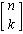, where the first is slightly easier to type.

For example, the list {1, 2, 3, 4} can be permuted into two cycles in the following ways:

• {{1,3,2}, {4}}
• {{1,2,3}, {4}}
• {{1,4,2}, {3}}
• {{1,2,4}, {3}}
• {{1,2}, {3,4}}
• {{1,4,3}, {2}}
• {{1,3,4}, {2}}
• {{1,3}, {2,4}}
• {{1,4}, {2,3}}
• {{1}, {2,4,3}}
• {{1}, {2,3,4}}

There are 11 such permutations, thus s(4, 2) = 11.

Here are some diagrams showing the cycles for permutations of a list with five elements.

s(5, 1) = 24, that is, all 5 elements form 1 cycle: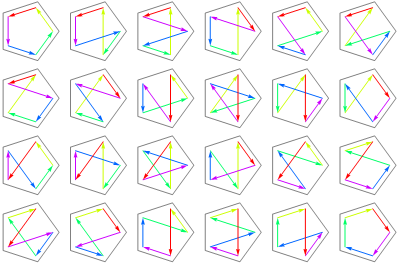s(5, 2) = 50, meaning 5 elements form 2 cycles, where a dot is a cycle of length 1: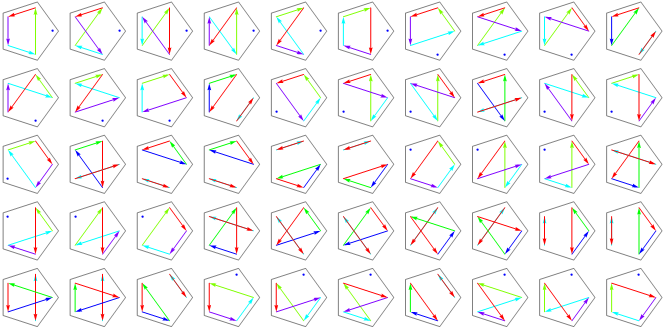s(5, 3) = 35: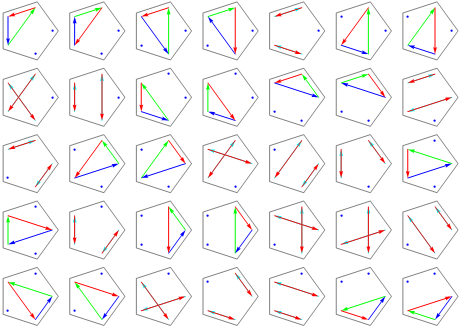s(5, 4) = 10: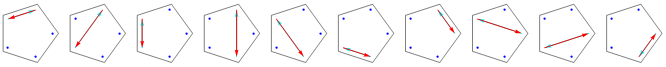s(5, 5) = 1: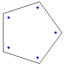Designed and rendered using Mathematica 3.0 (for NeXT) and—much later—7.0 (for Windows).

© 1996–2019 by Robert Dickau.

[ home ] || [ 2010-12-29 ]

www.robertdickau.com/stirling1.html